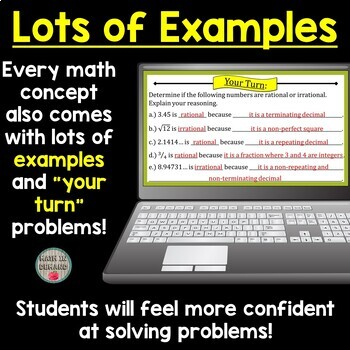### 8th Grade Math PowerPoints (Entire Year PRE-ORDER)

Included is a PRE-ORDER for an entire years worth of 8th Grade Math Editable PowerPoints. Currently, unit 1 is completed and ready to download! You will receive units 2 thru 5 by the dates listed below (or sooner!). You will not be charged an additional fee for the remaining units.Each PowerPoint includes animations! All you need to do is open the PowerPoint and click F5 to start the PowerPoint! There is a total of 47 PowerPoints.Each PowerPoint is aligned to my popular 8th Grade Math Interactive Notebook! However, you can use the PowerPoints without the notebook.The PowerPoints Include:

✅ The Real Number System

✅ Rationals vs Irrationals

✅ Converting a Fraction into a Repeating Decimal

✅ Converting a Repeating Decimal into a Fraction

✅ Square Roots and Cube Roots

✅ Approximating Non-Perfect Squares

✅ Non-Perfect Squares on the Number Line

✅ Comparing Irrational Numbers

✅ Exponent Properties

✅ Intro to Scientific Notation

✅ Multiplying/Dividing with Scientific Notation

Unit 2 - Expressions & Equations (UPLOADED BY 9/8/23)

✅ Unit Rate on a Graph

✅ Solving Equations with Variables on Both Sides

✅ Equations with Parentheses

✅ Number of Solutions to Linear Equations

✅ X and Y intercepts from a Table and Graph

✅ X and Y intercepts from an Equation

✅ Graphing using Intercepts

✅ Types of Slope

✅ Determining Slope Given a Graph

✅ Determining Slope Given a Table or Two Points

✅ Slope-Intercept Form

✅ Writing Linear Equations from a Graph

✅ Writing Linear Equations Given Two Points

✅ Standard Form to Slope-Intercept Form

✅ Types of Solutions from a Systems of Equations

✅ Solving Systems of Equations by Graphing

✅ Solving Systems of Equations by Substitution

✅ Solving Systems of Equations by Elimination

✅ Solving Systems of Equations Word Problems

Unit 3 - Functions (UPLOADED BY 9/22/23)

✅ Intro to Functions

✅ Function Notation and Evaluating a Function

✅ Linear vs Non-Linear

✅ Writing Linear Functions from a Table

✅ Comparing Functions (Graph, Table, Equation, and Verbal Description)

Unit 4 - Geometry (UPLOADED BY 10/6/23)

✅ Intro to Transformations

✅ Rigid Transformations: Reflections, Rotations, & Translations Rules

✅ Dilations

✅ Sequence of Transformations

✅ Similarity

✅ Congruence

✅ Identifying Angle Relationships

✅ Solving with Angle Relationships

✅ Triangle Angle Sum Theorem

✅ Triangle Exterior Angle Theorem

✅ Proving the Pythagorean Theorem

✅ Applying the Pythagorean Theorem

✅ Pythagorean Theorem in Coordinate System

✅ Volume: Cone, Cylinder, and Sphere

Unit 5 - Statistics & Probability (UPLOADED BY 10/20/23)

✅ Intro to Scatter Plots

✅ Constructing Scatter Plots

✅ Line of Best Fit

✅ Two Way TablesWant to purchase both my PowerPoints and Notebook? Click HERE for the bundle.

Related Products

This resource includes a limited use license from Math in Demand. You may only use the resource for personal classroom use. This purchase does not allow you to transfer my resource to another teacher, school, or etc. You must purchase an additional license at a discounted cost.

Questions? Please email me at mathindemand@hotmail.com or click the Q&A tab.